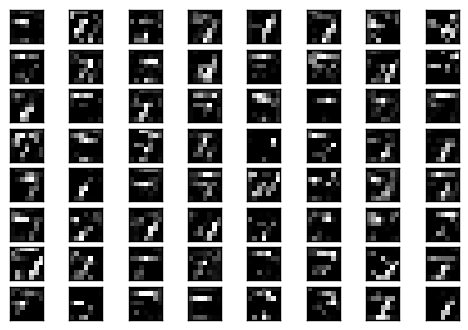#2019 iT 邦幫忙鐵人賽DAY 29
0

## [第29天]　卷積神經網絡　MNIST練習(4)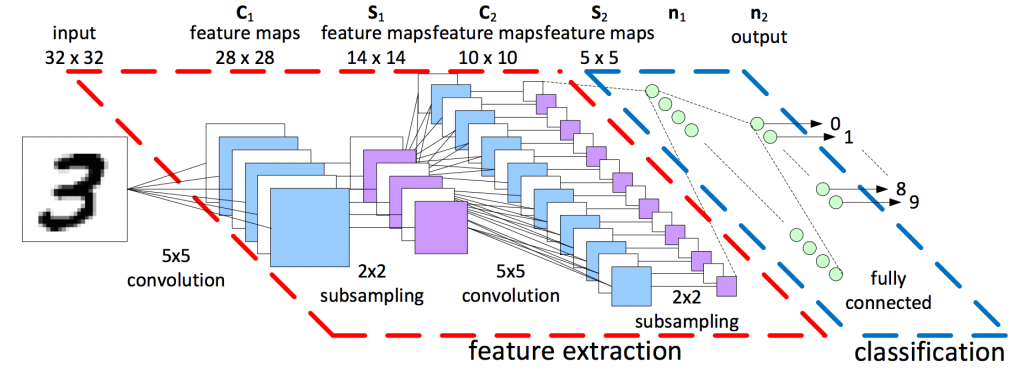參考網站1

``````# 印出第二層的filter
plot_conv_weights(W_conv2, 64)
``````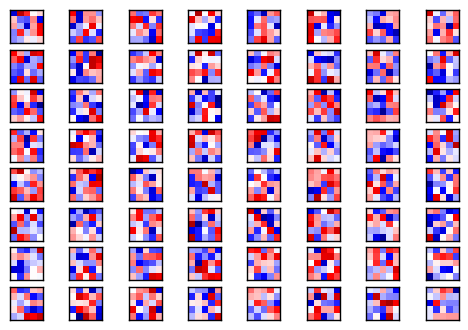``````# 第二層filter後的結果
plot_conv_layer(conv2d(h_pool1, W_conv2), mnist.test.images, 64)
``````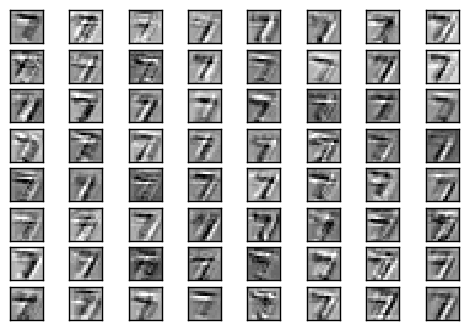``````# 第二層經過 ReLU 的結果
plot_conv_layer(tf.nn.relu(conv2d(h_pool1, W_conv2) + b_conv2), mnist.test.images, 64)
``````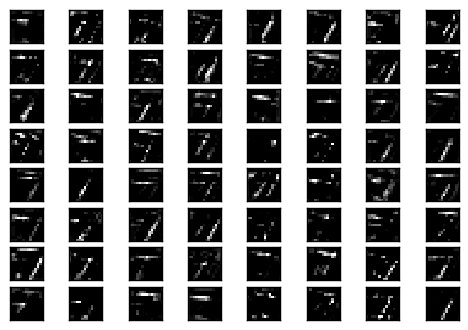``````# 第二層經過 MaxPooling 的結果
plot_conv_layer(max_pool_2x2(tf.nn.relu(conv2d(h_pool1, W_conv2) + b_conv2)), mnist.test.images, 64)
``````